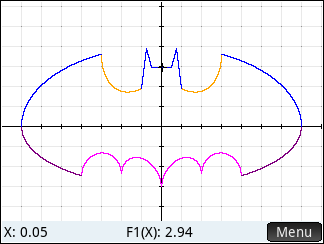Batman Meets HP Prime
01-29-2019, 11:39 AM
Post: #1
 mark4fliesMember Posts: 115 Joined: Jan 2015
Batman Meets HP Prime
From WolframAlpha: Batman.

Nice addition to the example library in the Advanced Graphing app, eh?
01-29-2019, 06:59 PM
Post: #2Jurgen KellerMember Posts: 297 Joined: Dec 2013
RE: Batman Meets HP Prime
For the lazy one's just open the Function app and copy the following formulas into F1(X) .. F4(X):

2*sqrt(-abs(abs(X)-1)*abs(3-abs(X))/((abs(X)-1)*(3-abs(X))))(1+abs(abs(X)-3)/(abs(X)-3))sqrt(1-(X/7)^2)+(5+0.97(abs(X-.5)+abs(X+.5))-3(abs(X-.75)+abs(X+.75)))(1+abs(1-abs(X))/(1-abs(X)))

-3*sqrt(1-(X/7)^2)sqrt(abs(abs(X)-4)/(abs(X)-4))

abs(X/2)-0.0913722(X^2)-3+sqrt(1-(abs(abs(X)-2)-1)^2)

(2.71052+(1.5-.5abs(X))-1.35526sqrt(4-(abs(X)-1)^2))sqrt(abs(abs(X)-1)/(abs(X)-1))+0.9

The resulting display is:It’s not who I am underneath, but what I do that defines me. – Batman
01-30-2019, 10:42 PM
Post: #3
 KomanguyJunior Member Posts: 49 Joined: Jul 2018
RE: Batman Meets HP Prime
(01-29-2019 06:59 PM)Jurgen Keller Wrote:  For the lazy one's just open the Function app and copy the following formulas into F1(X) .. F4(X):

2*sqrt(-abs(abs(X)-1)*abs(3-abs(X))/((abs(X)-1)*(3-abs(X))))(1+abs(abs(X)-3)/(abs(X)-3))sqrt(1-(X/7)^2)+(5+0.97(abs(X-.5)+abs(X+.5))-3(abs(X-.75)+abs(X+.75)))(1+abs(1-abs(X))/(1-abs(X)))

-3*sqrt(1-(X/7)^2)sqrt(abs(abs(X)-4)/(abs(X)-4))

abs(X/2)-0.0913722(X^2)-3+sqrt(1-(abs(abs(X)-2)-1)^2)

(2.71052+(1.5-.5abs(X))-1.35526sqrt(4-(abs(X)-1)^2))sqrt(abs(abs(X)-1)/(abs(X)-1))+0.9

The resulting display is:It’s not who I am underneath, but what I do that defines me. – Batman

yeah! great!

Guy R. KOMAN, hp 50G, hp Prime Rev. C
 « Next Oldest | Next Newest »

User(s) browsing this thread: 1 Guest(s)